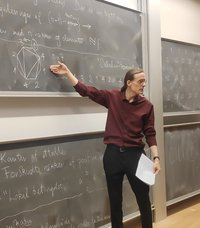Department of MathematicsOngoing research projects

Mathematical research still focuses primarily on the areas algebra, analysis and algebraic topology. Almost all mathematics staff are specialized within one of these main areas. Research activities increasingly focus on tasks related to more than one of these disciplines and to other areas, including application of mathematics within physics, computer science, genetics, economics etc. The Department is particularly active within research on: Algebraic K-theory and geometry of manifolds, algebraic geometry, Lie algebras and their representations, quantum algebras, category theory, homotopy theory, topology of smooth germs, dispersal theory, convexity theory, operator algebras, Bose algebras, combinatorics, algebraic number theory, representation of Lie groups, coding theory, cryptology, invariants of 3-dimensional manifolds and Gauge theory.

Research also includes singularity theory, topological quantum field theory, moduli space, mapping-class groups, computational biology, partial differential equations relating to mathematical physics, including scattering theory, super conductivity, operator theory and quantum mechanics, and analysis of manifolds. Research also revolves around harmonic analysis, analytical number theory, Diophantine analysis, K-theory for operator algebras and dynamical systems.

Research within theoretical statistics concentrates on probability theory and basic concepts of statistical inference, particularly stochastic analysis, Markov processes and associated potential theory, probability theory in infinite-dimensional space, actuarial mathematics and mathematical financing, simulation, asymptotic likelihood theory, inference for small random samples and stochastic processes, Edgeworth – and saddle point approximation, advanced statistical DNA models, time series analysis, geometric probability and stereology, spatial statistics, relationship between stochastic processes and quantum physics, analysis of concrete statistical models.

Research also includes stochastic geometry and bio imaging, space-time modeling and statistical methods within bioinformatics.

Operations research concentrates on optimization, optimal control theory, production economics and financing. The optimization theory concentrates on stochastic programming, multicriteria optimization and combinatorial optimization problems. Within production economics the application of Markov decision processes for stock control and pricing are analysed. Central topics within financing theory encompass pricing of contingent claims, theory of interest rates structure and the interaction between finance theory and actuarial science. Models to be applied within the energy sector are also analysed.

Comprehensive and externally financed centres and projects

Centre for Science Studies (CSS)

The aim of the Centre for Science Studies is to do:

• Research in the fields of history and philosophy of science and technology, science and society, and science communication
• Research-based development of education and communication activities

Homepage: css.au.dk

Center for Research in Econometric Analysis of Time Series (CREATES)

This centre is is a research unit at Aarhus BSS, hosted by the Department of Economics and Business Economics. Faculty of Natural Science. Researchers of the Institute of Mathematical Sciences specializing in financing participate in time series and financial econometrics research performed in CREATES.

The centre was established in 2007 by the Danish National Research Foundation (Centers of Excellence) with a grant of DKK 40 million.

Homepage: creates.au.dk

Sapere Aude - Semi Classical Quantum Mechanics

The project Semiclassical Quantum Mechanics is funded by a Sapere Aude: DFF-Topresearcher Grant from the Danish Council for Independent Research with Søren Fournais as Principal Investigator. In this project research is carried out in the area of mathematical quantum mechanics, in particular, spectral problems in the presence of magnetic fields. When the magnetic field is very strong and/or when many interacting particles are considered, one can often show that the quantum mechanical systems can be described by simpler - sometimes even classical - models. This can be seen as an aspect of the Correspondence Principle by Niels Bohr.

Homepage: Semiclassical Quantum Mechanics

Aarhus Homological Algebra

The Aarhus Homological Algebra Group is funded by a DNRF Chair and by Aarhus University Research Foundation.  Homological algebra is based on tools originally used to study topological spaces.  Its methods have spread to many areas of mathematics, including combinatorics, geometry, and mathematical physics.  Homological methods have become key instruments of the mathematical mainstream, and the Aarhus Homological Algebra Group is pursuing a vigorous research programme. There are applications to the combinatorics of high dimensional structures, the geometry of singular spaces, and the string theory associated to Calabi-Yau categories.Peter Jørgensen, photo: Kathrine Tang Riewe.